Next: Harmonic Ratios and Involutions Up: Some Standard Cross Ratios Previous: Projective Bases for the

## Cross Ratios of Planes

A pencil of planes in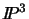is a family of planes having a common line of intersection. The cross ratio of four planes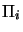of a pencil is the same as the cross ratio of the lines li of intersection of the planes with fifth, transversal plane (see fig. 3.3).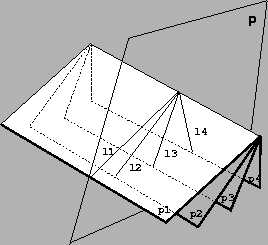Once again, different transversal planes give the same cross ratio as the figures they give are projectively equivalent. The Möbius formula also extends to this case: let P, Q be any two distinct points on the axis of the plane pencil, and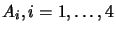be points lying on each plane(not on the axis), then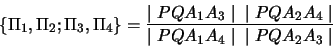where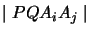stands for a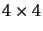determinant of 4-component column vectors.

Bill Triggs
1998-11-13